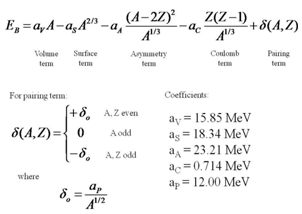# The Liquid Drop Model

## I-Tso Chen February 13, 2011

### Submitted as coursework for Physics 241, Stanford University, Winter 2011Fig. 1: The nuclear notation showing the chemical symbol, the mass number and the atomic number of the isotope. 

The liquid drop model, developed from the observation of similar properties between a nucleus and a drop of incompressible fluid, helps explain nuclear phenomena such as the energetic of nuclear fission and the binding energy of nuclear ground levels which cannot be illustrated by the shell model.  In view of similarities such as the latent heat of vaporization of fluid which is comparable to the constant binding energy per nucleon, and the surface tension effects of nucleus as well as a liquid drop, the quantitative aspect of the model delivers a formula that approximates the mass and binding energy of nuclei. 

More specifically, heat of vaporization represents the amount of energy required to convert molecules from liquid phase to gas phase. The latent heat of vaporization is proportional to the number of molecules in the liquid. The binding energy of nucleus displays a similar relationship where it is proportional to the number of nucleons. Using such analogy, the semi-empirical mass formula (also known as the Bethe-Weizaecker formula), was derived from the liquid drop model empirically as a function of mass number A and atomic number Z as shown in Figs. 1 and 2.Fig. 2: The semi-empirical mass formula with coefficients (±10% error) determined experimentally. 

There are five factors that contribute to the binding energy of nuclei. The volume term, being directly proportional to the number of nucleons, illustrates the idea that each nucleon only interacts with its nearest neighbors and binds to the nucleus at a specific binding energy. The surface term suggests that fewer nucleon interactions are observed on surface compared to that of nucleus interior, which is analogous to the surface tension of liquid drop. The Coulomb term represents the electrostatic repulsion between protons in a nucleus. The asymmetry term accounts for the difference in the number of protons and neutrons in the nuclear matter. The pairing term corrects for the coupling effects of protons and neutrons. 

One application of binding energy calculation is to determine the possibility of beta decay. In order for beta decay to occur, the binding energy of the final nucleus has to be higher than that of the original. Taking Selenium-82 (A=34) and Krypton-82 (A=36) as an example, the binding energy is approximately -277 MeV and 214 MeV respectively, calculated from the semi-empirical mass formula above. The decay of Selenium-82 into Krypton-82 is in fact double beta decay where two electrons are emitted in the process. 

© I-Tso Chen. The author grants permission to copy, distribute and display this work in unaltered form, with attribution to the author, for noncommercial purposes only. All other rights, including commercial rights, are reserved to the author.

## References

 S. Sharma, Atomic and Nuclear Physics (Dorling Kindersley, 2008).

 T.C. Upadhyay, Introduction to Modern Physics (Anmol Publications Pvt. Ltd., 1999).

 F. D. Mackie and G. Baym, Compressible Liquid Drop Nuclear Model and Mass Formula," Nucl. Phys. A 285, 332 (1977).

 C.B. Sampson, Ed., Textbook of Radiopharmacy: Theory and Practice (Gordon and Breach, 1994).

 K. Heyde, Basic Ideas and Concepts in Nuclear Physics (Institute of Physics, 2004).

 M. W. Kirson, "Mutual Influence of Terms in a Semi-Empirical Mass Formula," Nucl. Phys. A 798, 29 (2007).